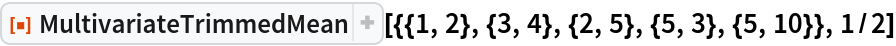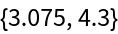Function Repository Resource:

# MultivariateTrimmedMean

Find the mean of multivariate data after removing outermost points

Contributed by: Wolfram Research
 ResourceFunction["MultivariateTrimmedMean"][matrix,f] gives the mean of the bivariate data matrix after dropping a fraction f of the outermost vectors.

## Details and Options

ResourceFunction["MultivariateTrimmedMean"] gives a robust estimate of the mean by excluding extreme values.
The outlying vectors are removed by repeatedly peeling off layers of convex hulls from the data until at least a fraction f have been removed.
ResourceFunction["MultivariateTrimmedMean"] interpolates between the means of the points remaining before and after the last layer is removed.
ResourceFunction["MultivariateTrimmedMean"][matrix,0] is equivalent to Mean[matrix].
ResourceFunction["MultivariateTrimmedMean"][matrix,f] approaches ConvexHullMedian[matrix] as f approaches 1.

## Examples

### Basic Examples (1)

MultivariateTrimmedMean of bivariate data:

 In:=Out=## Requirements

Wolfram Language 11.3 (March 2018) or above

## Version History

• 1.0.0 – 20 February 2019